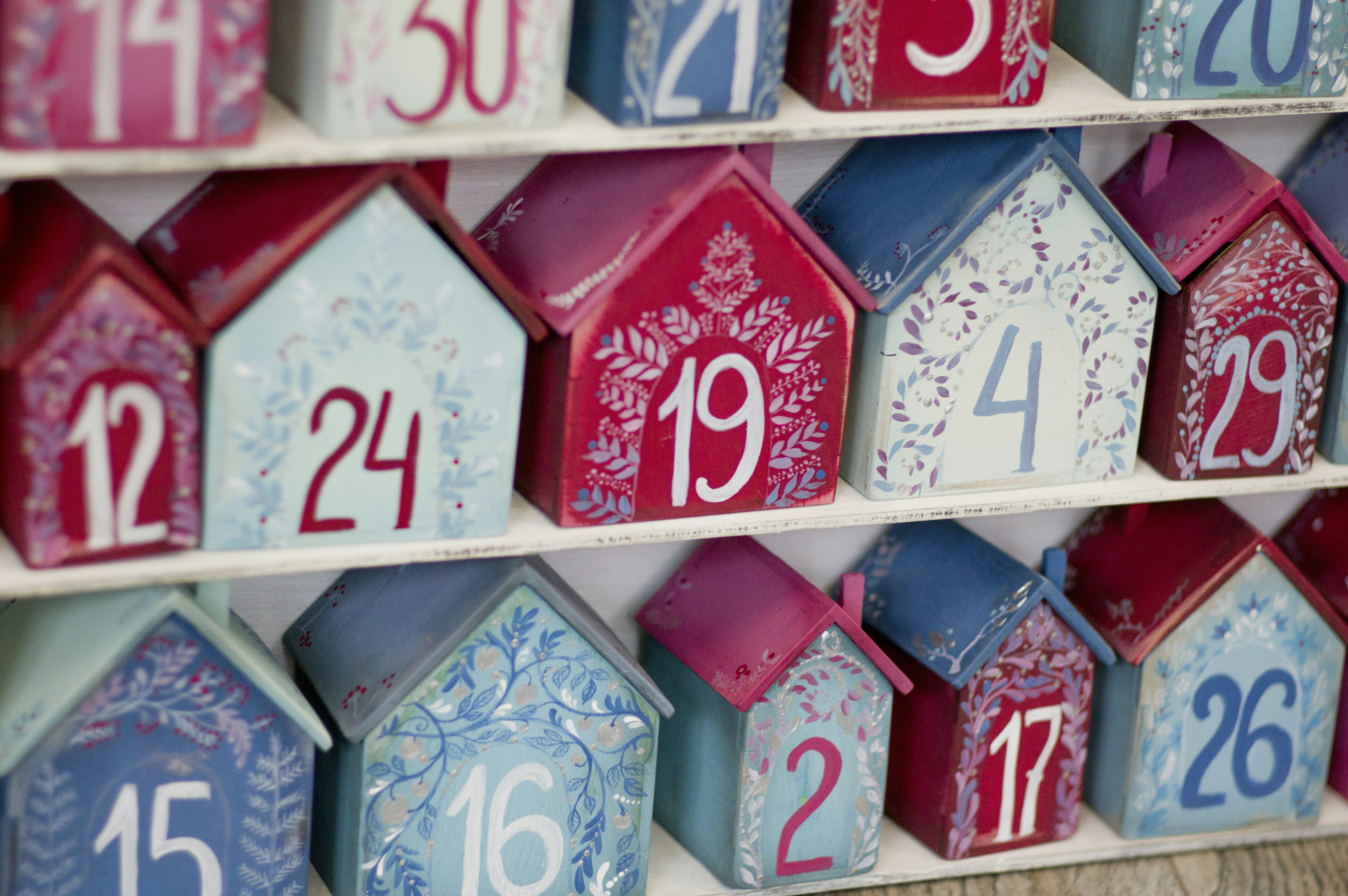# Advent of Code 2021 - Day 1

The advent of code is an annual coding challenge in the month of December. This article covers the first day's puzzle with a solution written in Go.Over the last few years, Advent of Code has gained a lot of popularity. Many developers use it as a creative way to challenge themselves or practice their skills in new programming languages. Nearly 200k people completed the first day this year.

Myself, I'm using this year to learn and practice Go. While I was one of the early adopters of Go in 2009 and used it for about a year during my postgraduate studies, I have not touched it since, since my career took me in a different direction. With the rising popularity of Kubernetes and microservices, Go seems like an excellent addition to my toolset.

I'll attempt to cover all of this year's challenges and explain my thought process going into them. Since the later exercises usually take a lot of time to solve, I expect this series to run well into 2022.

### Part 1

As per usual, the first day starts relatively easily. We're in a submarine with a sonar sweeping the ocean floor and recording the depth at certain intervals. The first task is to count the number of times a depth measurement increases.

We first need to read our puzzle input which is given as a sequence of positive integers with each number on a new line.

func loadMeasurements() []int {
if err != nil {
panic(err)
}

lines := strings.Split(string(bytes), "\n")

nums := []int{}
for _, val := range lines[:len(lines)-1] {
num, _ := strconv.Atoi(val)
nums = append(nums, num)
}

return nums
}

This snippet gives us an int-slice with all numbers from the file. It's now trivial to loop over this slice and count the number of increments:

var count, last int

last = measurements
for _, num := range measurements {
if num > last {
count++
}
last = num
}
fmt.Printf("Number of increments: %d\n", count)

This solves part 1.

### Part 2

We've now concluded that looking at every single measurement isn't useful, so we decide to instead look at the last three measurements. The goal is still the same, count the number of times the sum of three measurements is bigger than the three a measurement ago.

To visualize this a little bit, imagine the numbers are: 1 2 3 4 5 6. In this example the sum of the first three measurements 1 2 3 is 6. In the next step, we're looking at 2 3 4 whose sum is 9. This continues until we've run out of numbers.

The code for this is fairly simple to write. Start with the first three measurements and then step through the entire slice one by one:

count = 0
prev := math.MaxInt
for i := 0; i < len(measurements)-2; i++ {
sum := measurements[i] + measurements[i+1] + measurements[i+2]
if sum > prev {
count++
}
prev = sum
}

It's important to recognize that we want to ignore the first measurement, and we can do this by initializing prev to math.MaxInt since it ensures that our first measurement can't be bigger than prev.

### Summary

That's it for day 1. No crazy algorithms yet, but enough to prepare you for reading the problem descriptions and doing basic IO.

💡
If you like articles like this one, please consider subscribing to my free newsletter where at least once a week I send out my latest work covering Julia, Python, Machine Learning, and other tech.